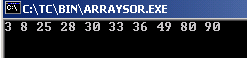Tutorials

# C array sort example

For sorting an array, we have used the qsort function. This function provides the implementation of quicksort algorithm to sort the elements of an array.

For sorting an array, we have used the qsort function. This function provides the implementation of quicksort algorithm to sort the elements of an array.

# C array sort example

In this section, you will learn how to sort an array in C.

For sorting an array, we have used the qsort function. This function provides the implementation of quicksort algorithm to sort the elements of an array.

Syntax of the function:
qsort(array, sizeof(array), sizeof(type), comparison_fn).

In the example, we have created a comparison function sort to compare two elements. For this we have passed two parameters (x and y ) which are pointers to elements and will return an int value by comparing them. This method compares each pair of elements. This function can be declared as:
int sort (const void * x, const void * y );

(*(int*)x - *(int*)y)- The parameters x and y  checks for each pair of elements considering the elements as x and y. If x is found greater than y, then x goes before y otherwise, x goes after y. In case if x = y, then it remains on the same position.

<stdlib.h> - This header file stands for standard library which includes functions involving String, Memory, Environment, Sorting and Searching, Math and Multibyte

Here is the code:

ARRAYSOR.C

 ``` #include  #include  #include  int array[] = { 90, 3, 33, 28, 80, 49, 8, 30, 36, 25 }; int sort(const void *x, const void *y) {   return (*(int*)x - *(int*)y); } void main() {   clrscr();   int i;   qsort(array, 10, sizeof(int), sort);   for (i=0; i<10; i++) {   printf("%d ", array[i]);   }   getch(); }```

Output will be displayed as:

ARRAYSOR.EXE